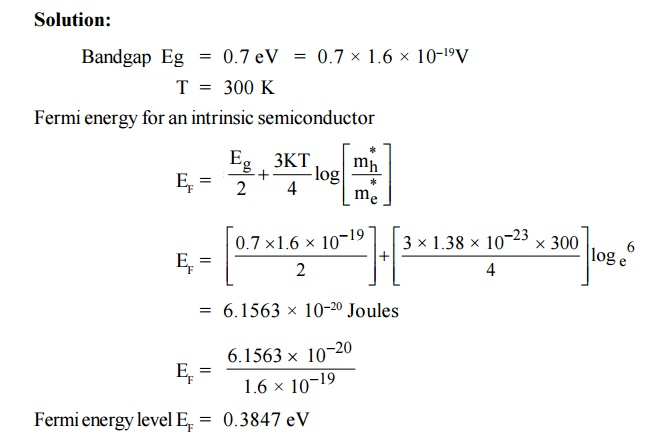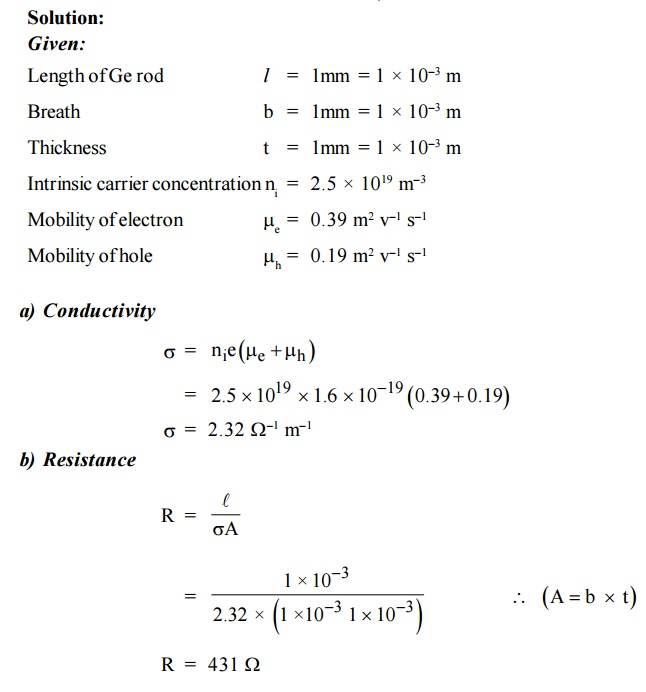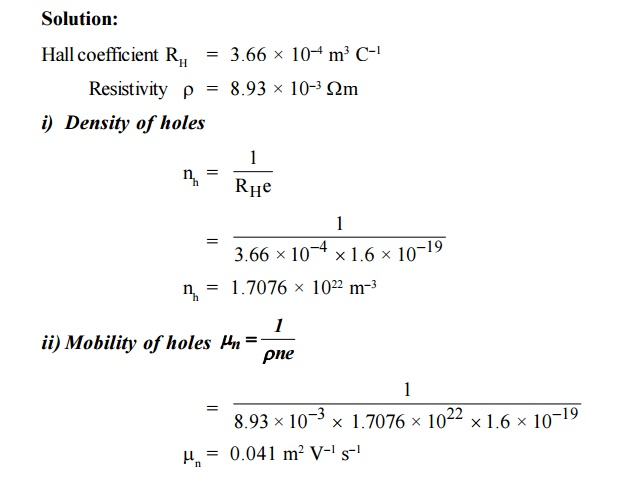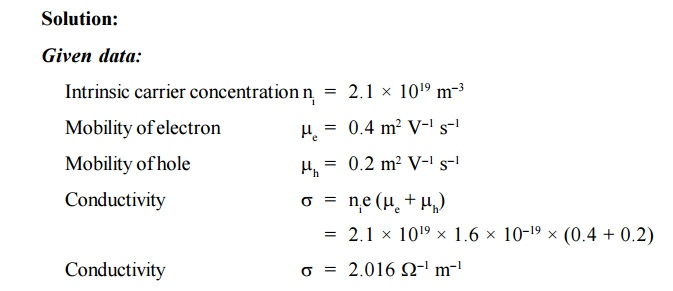Home | | Engineering Physics II | Solved Problems: Semiconducting Materials

# Solved Problems: Semiconducting Materials

Physics - Semiconducting Materials - Important Solved Problems(Sum): Semiconducting Materials

SOLVED PROBLEMS

1.Calculate the intrinsic concentration of charge carriers at 300 K given that m *e =0.12m o ,m *h =0.28mo and the value of brand gap = 0.67 eV. Solution:

Given:2.The intrinsic carrier density is 1.5 × 1016 m–3. If the mobility of electron and hole are 0.13 and 0.05 m2 V–1 s–1, calculate the conductivity.3.         The Intrinsic carrier density at room temperature in Ge is 2.37 × 1019 m3 if the electron and hole mobilities are 0.38 and 0.18 m2 V–1 s–1 respectively, calculate the resistivity.4.The Hall coefficient of certain silicon specimen was found to be –7.35 × 10–5 m3 C–1 from 100 to 400 K. Determine the nature of the semiconductor. If the conductivity was found to be 200 –1 m–1. Calculate the density and mobility of the charge carrier.

Solution:5. In a P-type germanium, ni = 2.1 × 1019 m–3density of boran 4.5 × 1023 atoms /m3. The electron and hole mobility are 0.4 and 0.2 m2 v–1 s–1 respectively. What is its conductivity before and after addition of boron atoms.6. An N-type semiconductor has hall coefficient = 4.16 × 10–4 m3 C–1. The conductivity is 108 –1 m–1. Calculate its charge carrier density ‘ne’and electron mobility at room temperature.

Solution:

Given:7. In an N-type semiconductor, the concentration of electron is 2 × 1022 m–3 . Its electrical conductivity is 112 –1 m–1. Calculate the mobility of electrons.8. For an intrinsic Semiconductor with a band gap of 0.7 eV, determine the position of EF at T = 300 K if m*h = 6m*e.9.A semiconducting crystal with 12 mm long, 5 mm wide and 1 mm thick has a magnetic density of 0.5 Wbm–2 applied from front to back perpendicular to largest faces. When a current of 20 mA flows length wise through the specimen, the voltage measured across its width is found to be 37μV . What is the Hall coefficient of this semiconductor?

Solution:

Given:10. Find the resistance of an intrinsic Ge rod 1 mm long, 1 mm wide and 1 mm thick at 300 K. the intrinsic carrier density 2.5 ×1019 m–3 is at 300 K and the mobility of electron and hole are 0.39 and 0.19 m2 v–1 s–1.11. Hall coefficient of a specimen of depend silicon found to be 3.66 × 10–4 m3 C–1. The resistivity of the specimen is 8.93 × 10–3 m. Find the mobility and density of the charge carriers.12. The intrinsic carrier density of a semiconductor is 2.1 × 1019 m–3. The electron and hole mobilities are 0.4 and 0.2 m2 V–1 s–1 respectively. Calculate the conductivity.

Solution:13. The electron mobility and hole mobility in Si are 0.135 m2 V–1 s–1 and 0.048 m2 V–1 s–1 respectively at room temperature. If the carrier concentration is 1.5 × 1016 m–3. Calculate the resistivity of Si at room temperature.ASSIGNMENT PROBLEMS

1.  Find the resistance of an intrinsic germanium rod 1 cm long, 1mm wide and 1mm thick at 300 K. the intrinsic carrier density is 2.5 × 1019 / m–3 at 300 K and the mobility of electron and hole are 0.39 and 0.19 m2 V–1 S–1. (Ans: 4.31 × 103)

Calculate the position of Fermi level EF and the conductivity at 300 K for germanium crystal containing 5 × 1022 arsenic atoms / m3. Also calculate the conductivity if the mobility of the electron is 0.39 m2 V–1 S–1.

(Ans : EF is 0.16 eV below Ec   = 3210 –1 m–1)

In a Hall experiment a current of 25 A is passed through a long foil of silver which is 0.1mm thick and 3cm wide. If the magnetic field of flux density 0.14 Wb/m2 is applied perpendicular to the foil, calculate the Hall voltage development and estimate the mobility of electrons in silver. The conductivity the Hall coefficient is (–8.4 × 10–11)m3 / coulomb. (Ans : 29.4 V and 57.7 × 10–4 m2 V–1)

The intrinsic carrier density at room temperature in Ge is 2.37 × 1019 m3. If the electron and hole motilities are 0.38 and 0.18 m2 V1 S1 respectively, calculate the resistivity.

(Ans : 0471   m)

5.  For silicon semiconductor with band gap1.12 eV, determine the position of the Fermi level at 300 K, if m*e 0.12m0 and m*h 0.28m0 (Ans : 0.576 eV)

For an intrinsic semiconductor with gap width Eg = 0.7 eV, calculate the concentration of intrinsic charge carriers at 300 K assuming that m*e m*h m0 .

(Ans : 33.49 × 1018 / m3)

A silicon plate of thickness 1mm, breadth 10mm, and length 100mm is placed magnetic field of 0.5 wb/m2 acting perpendicular to its thickness. If A 10–2 current flows along its length, calculate the Hall voltage developed if the Hall coefficient is 3.66 × 10–4 m3 / coulomb. (Ans : 3.7 × 106 C–1 m3)

A N-type semiconductor has Hall coefficient = 4.16 × 10–4 C–1 m3. The conductivity is 108 ohm–1 m–1. Calculate its charge carrier density and electron mobility at room temperature.    (Ans : 0.038 m2 V–1 S–1)

Study Material, Lecturing Notes, Assignment, Reference, Wiki description explanation, brief detail
Physics : Semiconducting Materials : Solved Problems: Semiconducting Materials |